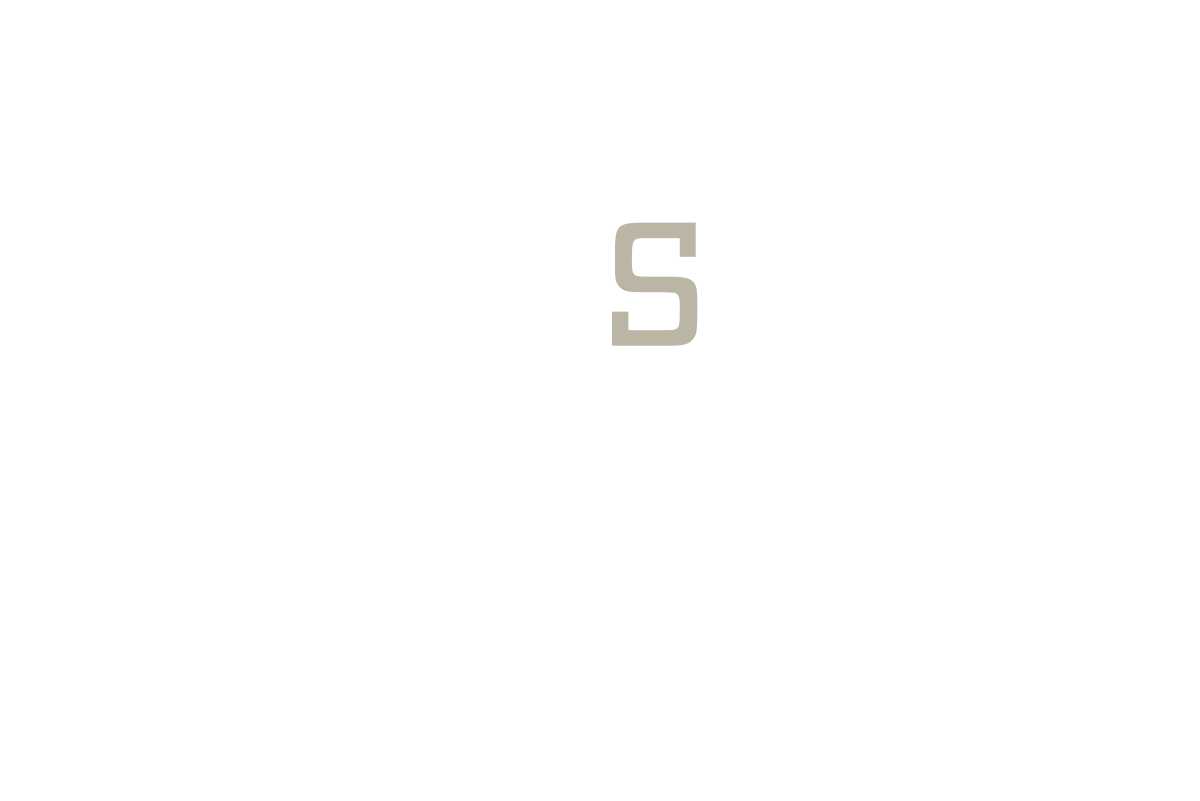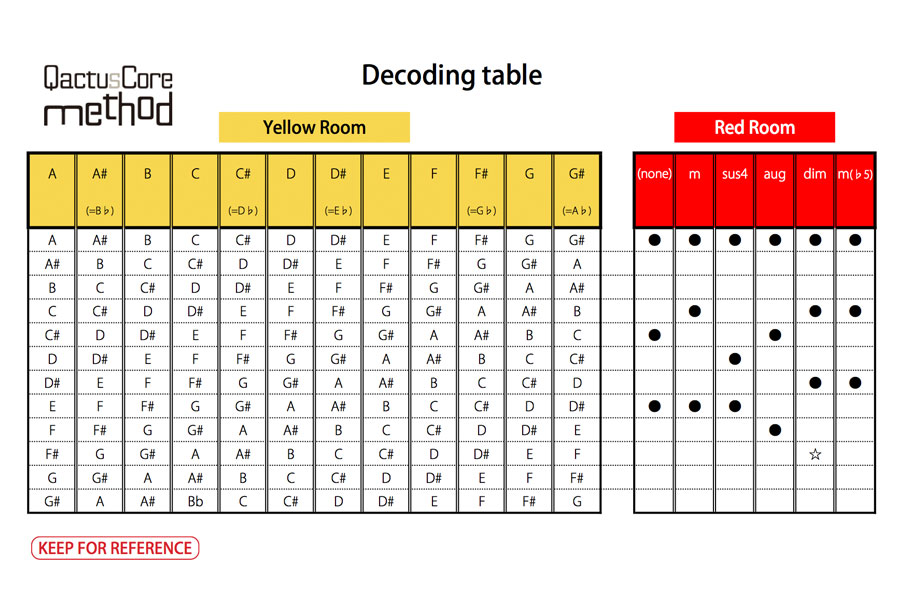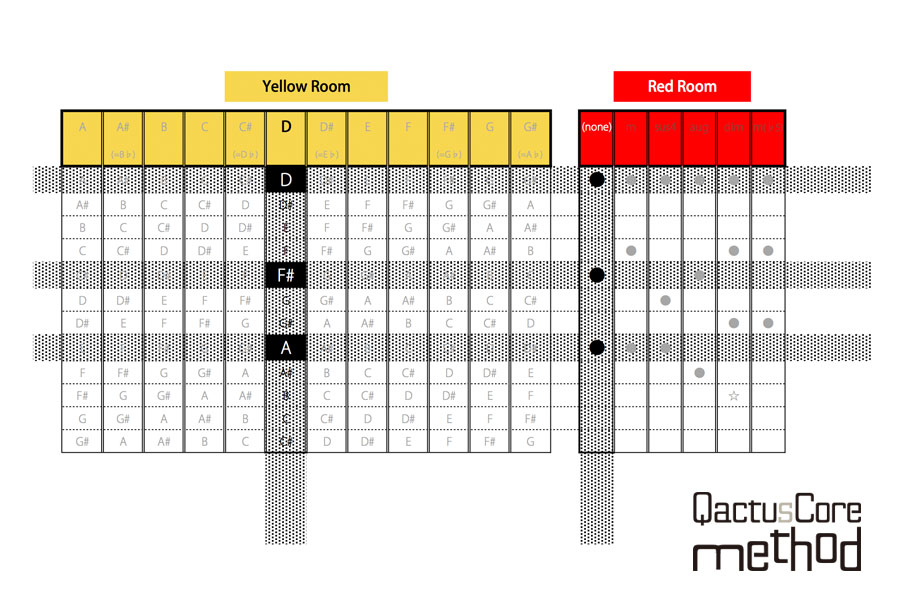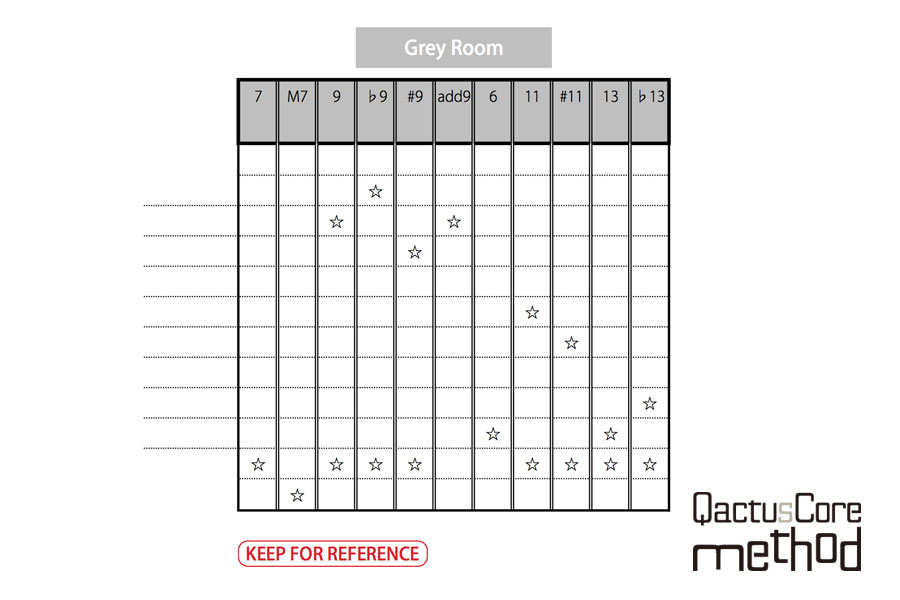01

02

04

pagetop

# STAGE-1

### 2. To Calculate Particular Musical Notes From Chords

Most chords are basically three notes.

The sound you’re playing is going to harmonize as long as these string notes belong to the chord constituent.

We can determine the musical notes from any chords if we have some knowledge related to music theory.

Don’t worry, we can calculate particular musical notes from chords with this chart "Decoding Table."We will learn how to use this decoding table.

First, pick a simple chord.

[Em] is a good example.

Use the decoding table to divide the chord into its harmonizing notes, and follow these steps:
1. Find [E] in The Yellow Room, (it’s the 8th column from the left).
2. Now find the [m] in The Red Room, (it’s the 2nd column from the left).
3. Use the decoding table to find the particular marks between the two rooms (Yellow and Red).
4. Match the marks in the row from The Red Room’s [m] column with the notes in the row of The Yellow Room’s [E] column.

Just to let you know, the correct answer of the sample [Em] is [E], [G], [B].

That’s it for now.
It’s easy if you can find the notes on each line carefully.

Let’s practice, OK?

Try to find particular notes that harmonize in these five chords.

Question
1. D
2. F#m
3. Bm7
4. Am(♭5)
5. Gdim

You may have noticed that there some challenging exercises in this set of chords. This is for you to practice so that you will be able to perfect it.

Let’s do these, in order.

Q1: D

The chart in The Yellow Room has [D] in the 6th column from the left. We need to choose the 1st column named [none] in The Red Room. [D] has no mark in The Red Room.

Now, let’s find the crossing points with the rows in The Yellow Room’s [D] column with the marks in the row from The Red Room’s [none] column.

[D], [F#], [A] are exactly the notes that harmonize with this chord.Q2. F#m

[F#], [A], [C#] are the notes that harmonize with the chord [F#m].

Now find [F#] in The Yellow Room and find [m] in The Red Room.
(Go back to [1. Chords & the 3 Rooms] to read again carefully if you are confused with this part)

[F#] is in the 10th column in The Yellow Room and [m] is in the 2nd column in The Red Room. Find the crossing points between the rows in The Yellow Room’s [F#] column and the marks in The Red Room’s [m] column.

Therefore, [F#], [A], [C#] are the notes that harmonize with the chord [F#m].

Q3: Bm7

[B], [D], [F#] are the notes that harmonize with the chord [Bm7].

This is a tricky question.

The Yellow Room has [B] and The Red Room has [m].
But where do we find the remaining  ?

Remember The Gray Room we told you to ignore? This is where we find ! In The Gray Room!
(Go back to [1. Chords & the 3 Rooms] to read again carefully if you are confused with this part.)

You can ignore  for now. Let’s see the crossing points between the rows in The Yellow Room’s [B] column and the marks in The Red Room’s [m] column.

Therefore, [B], [D], [F#] are the notes that harmonize with the chord [Bm7].

Q4: Am(♭5)

[A], [C], [D#] are the notes that can harmonize with the chord [Am(♭5)].

You don’t have to overthink it even if it looks like a tricky question.

Find [A] in The Yellow Room’s columns and find [m(♭5)] in The Red Room’s columns.

[m(♭5)] looks a bit weird compared to others, but it’s one of the options for The Red Room.

Now, let’s see the crossing points between rows in The Yellow Room’s [A]column and marks in The Red Room’s [m(♭5)] column.

Therefore, [A], [C], [D#] are the notes that can harmonize with the chord [Am(♭5)].

By the way, we have so many cases to add  onto this type of chords like [Am7(♭5)].  is  even if the chord is out of order.

It will be ok if you can imagine this kind of chord [Am(♭5)] with  removed.

Q5: Gdim

[G], [A#], [C#], [E] are the notes that harmonize with the chord [Gdim].

This kind of chord is much easier than we think. It’s simpe if you just divide it into [G] and [dim].
However, The Red Room has [☆]; so, actually, this is a tricky question.

Remember that [dim] is unlike other chords because it’s a bit special.
The four [G], [A#], [C#], [E] includes the [☆] and are the notes which can harmonize with the chord [Gdim].
It will still harmonizes if you remove [☆] or [E] to change [Gdim] into a three note chord.

You can ignore [☆] if you are confused.
It is much better than giving up.We are going to skip the explanation about this chart because we already learned how to use it based on how to use.
You are allowed to ignore [The Gray Room] to avoid confusion.

Now, we have learned how to find particular notes using this QactusCore-Method and the 3 Rooms.

pagetop